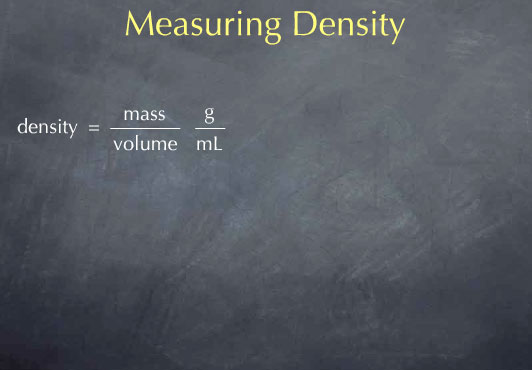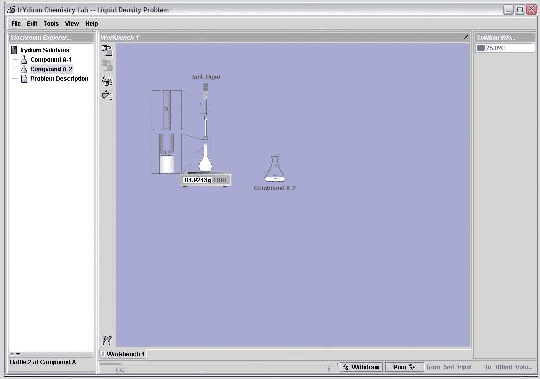# Stoichiometry Tutorials: Measuring Density

(from a complete OLI stoichiometry course)

The following video illustrates the concept of density and explains how density is measured.

### Measuring Density Movie Text

The density of a substance is defined as the mass of that substance per unit volume. To measure the density, I need to measure both the mass and volume of a sample. The ratio of the mass to the volume then gives me the density. If I were to measure the mass in grams and measure the volume in liters, I would get units of gram per liter for the density. A much more common unit for density, however, is to use milliliters for the volume, yielding the density in units of grams per milliliter. This is convenient because the density of liquid water is 1 g/mL at room temperature.Let's use the Virtual Lab to measure the density of a liquid, here labeled compound A-2. The lab is in realistic transfer mode, so I'll do this procedure as I would in a real lab. I need to measure the mass of the sample, so I'll pull out a scale. I also need to measure the volume, so I'll pull out a 100 mL volumetric flask. I put the flask on the scale and hit the tare button to zero the reading. I now need to put 100 mL of liquid into the flask. I begin by pouring directly from the erlenmeyer flask into the volumetric flask, until I get close to the 100 mL mark. I'll then use a 5 mL pipet to slowly transfer solution to the volumetric until the volume reaches 100 mL. Since I've now measured both the mass of the sample and its volume, I have what I need to determine the density.From the reading on the scale, I see that the mass of the sample is 84.924 grams. The volume of the sample is 100.0 mL. (A volumetric flask is accurate to about 0.1 mL, so I know the volume to 4 significant figures.) I divide the mass by the volume to get 0.8492 g/mL, the density of the liquid.Use this information to answer the question below.

The content above has been converted from Adobe Flash Player and may not display correctly.